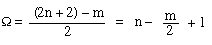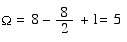### Spectroscopy Tutorial: Degree of Unsaturation

A calculation of the degree of unsaturation is a good way to start a spectroscopy problem. It tells you how many rings and double bonds are in the molecule; thus, you know if you should look for a carbonyl or a carbon-carbon double bond, or a ring or an aromatic ring.

The degree of unsaturation can be calculated readily from the molecular formula of all compounds containing carbon, hydrogen, oxygen, nitrogen, sulfur, or the halogens, by applying the following rules:

• Rule 1: Replace all halogens in the molecular formula by hydrogens.
• Rule 2: Omit oxygens and sulfurs.
• Rule 3: For each nitrogen, omit the nitrogen and one hydrogen.

Applications of these rules reduces the molecular formula in question to the molecular formula of the hydrocarbon which has the same degree of unsaturation. The degree of unsaturation of a hydrocarbon is easily deduced if one remembers that a saturated hydrocarbon has the formula CnH2n + 2. Thus for the formula CnHm,Applying these rules to the molecular formula C8H8NOBr.

• Rule 1, replace halogens with hydrogens: C8H9NO
• Rule 2, omit oxygens: C8H9N
• Rule 3, omit the nitrogen and one hydrogen: C8H8

Therefore,This means that the molecule has five rings and/or pi bonds (or any combination of the two). A degree of unsaturation greater than or equal to 4 doesn’t demand but should suggest the possibility of an aromatic (benzene) ring.

### More help:

Calculating Degrees of Unsaturation on the Organic Chemistry OnLine site.